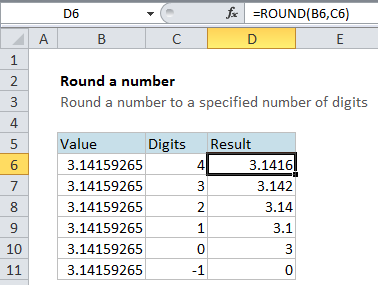Excel Round a number Example

If you need to round a number in a normal fashion (i.e. round values of 5 or more up and values less than 5 down) you can use the ROUND function with a specified number of digits.

This tutorials shows how to Round specific number in Excel.

Formula

`=ROUND(number,digits)`Explanation

In the example, the formula in cell D6 is

`=ROUND(B6,C6)`

This tells Excel to take the value in B6 (PI) and round it to the number of digits in cell C6 (4) with a result of 3.1416

Also See:   Excel Get integer part of a number Example

In the table, the ROUND function is used to round the same number (PI) to a decreasing number of digits, starting at 4 and moving down past zero to -1.

Notice that positive numbers round to the right of the decimal point, while digits specified less than or equal to zero round to the left.

Also See:   Excel Rank race results Example